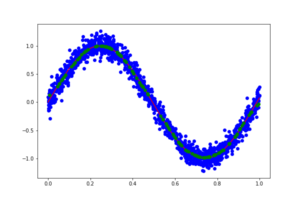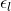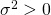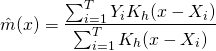# Kernel Regression using Pyspark

## 1. Kernel Regression using PysparkThe red curve shows the true function m(x) while the green dots show the estimated curve evaluated using an random grid. The blue points are the simulated . A well known problem of the estimation method concerning boundary points is clearly visible.

In a previous article I presented an implementation of a kernel denisty estimation using pyspark. It is thus not difficult to modify the algorithm to estimate a kernel regression. Suppose that there exits some function, an example for such functions are for instance temperature curves which measure the temperature during a day. In practice, such functionswill often not be directly observed, but one will have to deal with discrete, noisy observations contaminated with some error. The purpose of kernel regression is then to estimate the underlying true function.

In the following I will only consider a simple, standard error model: Fordesign pointsthere are noisy observationssuch that

(1)for i.i.d. zero mean error termswith finite varianceand.

To estimateI stick to the Nadaraya-Watson-Estimator given bywhereis a kernel as described here, in the implementation again an Epanechnikov kernelis used. The reader might recognize thatis just the density (scaled by) already implemented here, whileis just a weighted version of it. Implementation should therefore be very similar leading to the following algortihm:

###A Spark-Function to derive a non-parametric kernel regression
from pyspark.mllib.regression import LabeledPoint
from pyspark.mllib.feature import StandardScaler
import matplotlib.pyplot as plt
from numpy import *

##1.0 Simulated Data
T=1500

time=sort( random.uniform(0,1,T) )   ##Sorting is not required, i did it to have less trouble with the plots
true = sin( true_divide( time, 0.05*pi)  )
X= true + random.normal(0,0.1,T)

data= sc.parallelize(  zip(time,X)  )

plt.plot(time,true, 'bo')
plt.plot(time,X, 'bo')
plt.show()

##2.0 The Function
#2.1 Kernel Function

def spark_regression(data, Nout, bw):
def epan_kernel(x,y,b):
u=true_divide( (x-y), b)
return max(0, true_divide( 1, b)*true_divide(3,4)*(1-u**2))

#derive the minia and maxi used for interpolation
mini=data.map(lambda x: x).takeOrdered(1, lambda x: x )
maxi=data.map(lambda x: x).takeOrdered(1, lambda x: -1*x )
#create an interpolation grid (in fact this time it's random)
random_grid = sc.parallelize(  random.uniform(maxi,mini,Nout)  )
#compute K(x-xi) Matrix
density=data.cartesian(random_grid).map(lambda x:( float(x),epan_kernel(array(x),array(x),bw) ) )
kernl=data.cartesian(random_grid).map(lambda x:( float(x),x*epan_kernel(array(x),array(x),bw) ) )

mx= kernl.filter(lambda x: x&amp;gt;0).reduceByKey( lambda y, x:  y+x ).zip( density.filter(lambda x: x&amp;gt;0).reduceByKey( lambda y, x:  y+x ) )  ##added optional filter() does anyone know if this improves performance?
return mx.map(lambda x: (x,true_divide(x,x))  )

##3.0 Results

fitted= spark_regression(data, 128, 0.05).collect()
fit=array(fitted).transpose()

plt.plot(time,true, color='r')
plt.plot(fit, fit, 'bo')

plt.show()


### 1 thought on “Kernel Regression using Pyspark”

This site uses Akismet to reduce spam. Learn how your comment data is processed.